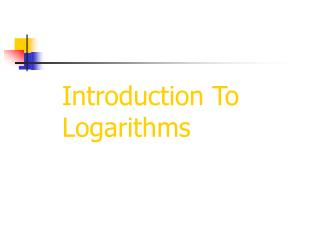DownloadDownload PresentationIntroduction To Logarithms

# Introduction To Logarithms

Télécharger la présentation## Introduction To Logarithms

- - - - - - - - - - - - - - - - - - - - - - - - - - - E N D - - - - - - - - - - - - - - - - - - - - - - - - - - -
##### Presentation Transcript

1. Introduction To Logarithms

2. Logarithms were originally developed to simplify complex arithmetic calculations. They were designed to transform multiplicative processes into additive ones.

3. If at first this seems like no big deal, then try multiplying 2,234,459,912 and 3,456,234,459. Without a calculator ! Clearly, it is a lot easier to add these two numbers.

4. Today of course we have calculators and scientific notation to deal with such large numbers. So at first glance, it would seem that logarithms have become obsolete.

5. Indeed, they would be obsolete except for one very important property of logarithms. It is called the power property and we will learn about it in another lesson. For now we need only to observe that it is an extremely important part of solving exponential equations.

6. Our first job is to try to make some sense of logarithms.

7. Our first question then must be: What is a logarithm ?

8. Of course logarithms have a precise mathematical definition just like all terms in mathematics. So let’s start with that.

9. Definition of Logarithm Suppose b>0 and b≠1, there is a number ‘p’ such that:

10. Now a mathematician understands exactly what that means. But, many a student is left scratching their head.

11. The first, and perhaps the most important step, in understanding logarithms is to realize that they always relate back to exponential equations.

12. You must be able to convert an exponential equation into logarithmic form and vice versa. So let’s get a lot of practice with this !

13. Example 1: Solution: We read this as: ”the log base 2 of 8 is equal to 3”.

14. Example 1a: Solution: Read as: “the log base 4 of 16 is equal to 2”.

15. Example 1b: Solution:

16. Okay, so now it’s time for you to try some on your own.

17. Solution:

18. Solution:

19. Solution:

20. It is also very important to be able to start with a logarithmic expression and change this into exponential form. This is simply the reverse of what we just did.

21. Example 1: Solution:

22. Example 2: Solution:

23. Okay, now you try these next three.

24. Solution:

25. Solution:

26. Solution:

27. We now know that a logarithm is perhaps best understood as being closely related to an exponential equation. In fact, whenever we get stuck in the problems that follow we will return to this one simple insight. We might even state a simple rule.

28. When working with logarithms, if ever you get “stuck”, try rewriting the problem in exponential form. Conversely, when working with exponential expressions, if ever you get “stuck”, try rewriting the problem in logarithmic form.

29. Let’s see if this simple rule can help us solve some of the following problems.

30. Solution: Let’s rewrite the problem in exponential form. We’re finished !

31. Solution: Rewrite the problem in exponential form.

32. Example 3 Solution: Try setting this up like this: Now rewrite in exponential form.

33. These next two problems tend to be some of the trickiest to evaluate. Actually, they are merely identities and the use of our simple rule will show this.

34. Example 4 Solution: First, we write the problem with a variable. Now take it out of the logarithmic form and write it in exponential form.

35. Example 5 Solution: First, we write the problem with a variable. Now take it out of the exponential form and write it in logarithmic form.

37. Finally, we want to take a look at the Property of Equality for Logarithmic Functions. Basically, with logarithmic functions, if the bases match on both sides of the equal sign , then simply set the arguments equal.

38. Example 1 Solution: Since the bases are both ‘3’ we simply set the arguments equal.

39. Example 2 Solution: Since the bases are both ‘8’ we simply set the arguments equal. Factor continued on the next page

40. Example 2 continued Solution: It appears that we have 2 solutions here. If we take a closer look at the definition of a logarithm however, we will see that not only must we use positive bases, but also we see that the arguments must be positive as well. Therefore -2 is not a solution. Let’s end this lesson by taking a closer look at this.

41. Our final concern then is to determine why logarithms like the one below are undefined. Can anyone give us an explanation ?

42. One easy explanation is to simply rewrite this logarithm in exponential form. We’ll then see why a negative value is not permitted. First, we write the problem with a variable. Now take it out of the logarithmic form and write it in exponential form. What power of 2 would gives us -8 ? Hence expressions of this type are undefined.

43. That concludes our introduction to logarithms. In the lessons to follow we will learn some important properties of logarithms. One of these properties will give us a very important tool which we need to solve exponential equations. Until then let’s practice with the basic themes of this lesson.